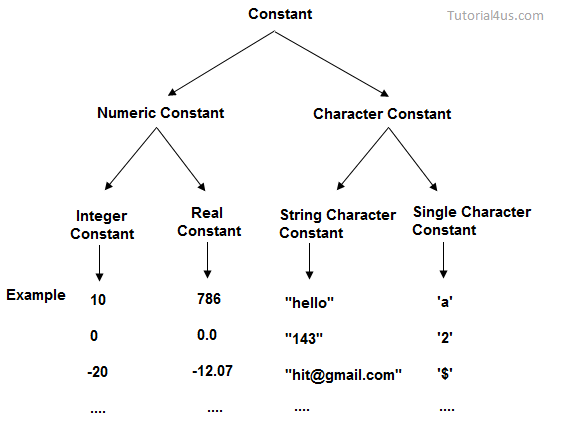# Constants or Literals in C?## Constants or Literals in C

Constants are the fixed values that never change during the execution of a program. Following are the various types of constants:• C Constants are also like normal variables. But, only difference is, their values can not be modified by the program once they are defined.
• Constants refer to fixed values. They are also called as literals
• Constants may be belonging to any of the data type.
• Syntax:

const data_type variable_name; (or) const data_type *variable_name;

## TYPES OF C CONSTANT:

1. Integer constants
2. Real or Floating point constants
3. Octal & Hexadecimal constants
4. Character constants
5. String constants
6. Backslash character constants
 Constant type data type (Example) Integer constants int (53, 762, -478 etc )unsigned int (5000u, 1000U etc)long int, long long int(483,647 2,147,483,680) Real or Floating point constants float (10.456789)doule (600.123456789) Octal constant int (Example: 013 /*starts with 0 */) Hexadecimal constant int (Example: 0x90 /*starts with 0x*/) character constants char (Example: ‘A’, ‘B’, ‘C’) string constants char (Example: “ABCD”, “Hai”)## 1. INTEGER CONSTANTS IN C:

• An integer constant must have at least one digit.
• It must not have a decimal point.
• It can either be positive or negative.
• No commas or blanks are allowed within an integer constant.
• If no sign precedes an integer constant, it is assumed to be positive.
• The allowable range for integer constants is -32768 to 32767.

## 2. REAL CONSTANTS IN C:

• A real constant must have at least one digit
• It must have a decimal point
• It could be either positive or negative
• If no sign precedes an integer constant, it is assumed to be positive.
• No commas or blanks are allowed within a real constant.

## 3. CHARACTER AND STRING CONSTANTS IN C:

• A character constant is a single alphabet, a single digit or a single special symbol enclosed within single quotes.
• The maximum length of a character constant is 1 character.
• String constants are  enclosed within double quotes.

## 4. BACKSLASH CHARACTER CONSTANTS IN C:

• There are some characters which have special meaning in C language.
• They should be preceded by backslash symbol to make use of special function of them.
• Given below is the list of special characters and their purpose.
 Backslash_character Meaning  Backspace f Form feed New line Carriage return Horizontal tab ” Double quote ’ Single quote \ Backslash v Vertical tab a Alert or bell ? Question mark N Octal constant (N is an octal constant) XN Hexadecimal constant (N – hex.dcml cnst)

## 1) C const keyword

The const keyword is used to define constant in C programming.

1. const float PI=3.14;

Now, the value of PI variable can t be changed.

 #include     int main(){         const float PI=3.14;         printf("The value of PI is: %f",PI);         return 0;   }

Output:

```The value of PI is: 3.140000
```

If you try to change the the value of PI, it will render compile time error.

 #include     int main(){     const float PI=3.14;      PI=4.5;     printf("The value of PI is: %f",PI);         return 0;   }

Output:

```Compile Time Error: Cannot modify a const object
```

## 2) C #define preprocessor

The #define preprocessor is also used to define constant. We will learn about #define preprocessor directive later.

Let s see an example of #define to create a macro.

 #include    #define MIN(a,b) ((a)<(b)?(a):(b))   void main() {      printf("Minimum between 10 and 20 is: %d ", MIN(10,20));     }